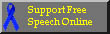WEEKDAY CALCULATOR
___________________________________________________________________________

 NAVIGATOR MAIN PAGE WHO AM I FILES THANK YOU SEARCH LINKS PERSONAL GUESTBOOK E-MAIL ME

 Here is an explanation how to calculate it: First subtract 1889, 1917, 1945, 1973, 2001 or any other year each 28th, from your year. 1968 - 1945 = 23 Then we must calculate leapyears. Divide the number you got with 4, do not care about the fraction-part. 23 / 4 = 5.75 But skip the fraction to get 5 Now this small number should be added to the other number (NOT the year) 23 + 5 = 28 Now we should remove 7 until the number is less than 7 (or in other words, remove 7, 14, 21, 35, 49 etc, the biggest that fits) 28 - 28 = 0 This you got is the YEAR-NUMBER If the original year is a leap-year, you have two year-numbers, the one we calculated for dates in January and February, and for the other months you should use the number added by one. The year 1968 IS a leap-year (dividable by 4) So the YEAR-NUMBER for March to December is 1 (This whole calculation is only valid for years 1901-2099 so dividable by 4 is enough for determining leap-year.) Now the month. The easiest is just to remember the series, 0 3 3 6 1 4 6 2 5 0 3 5 Each numer is for January (0), February(3) etc up to December (5). But why do we have theese numbers? It is simply the number of days MORE than even weeks in the PREVIOUS months. There are no month before January, therefore it has 0. January has 31 days, thats 3 more than even weeks (31-28=3), so February is 3 February is even 28 days (the leap-year we added above if needed) so March too is 3 March is 31 days, add this 3 extra days to the previous 3, and you get 6 for April. April has 2 days extra, 6 + 2 = 8, but again we take away 7 when it reaches that, so we get 1 for May. It continues the same for the rest of the months, to get the series mentioned above.So for Month 7 the MONTH-NUMBER is 6 Now we add the YEAR-NUMBER 1 and the MONTH-NUMBER 6 and the DAY 18 and we get 25 Again we take away 7 until it is below 7 (We could wait until now to take away any extra 7:s, but it's easier to do it at every point if one does not have a calculator). 25 - 21 = 4 The resulting number is the Weekday. 0=Sunday, 1=Monday etc and 6=Saturday. So... The weekday on (YYYY-MM-DD) 1968-7-18 is Thursday
What weekday are you born? Fill out this form and click to find out:
Year 1901-2099   Month   Day
___________________________________________________________________________